```
```

### Practical columns - design equations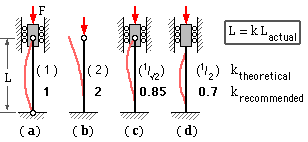An ideal column is perfectly straight initially, supported by pinned ends which are constrained to move axially, and loaded by a coaxial compressive force   F as shown in sketch ( a).

Illustrated also are other support arrangements which may be modelled by the configuration ( a) with an equivalent length   L related to the actual length of the arrangement by a factor   k which reflects the degree of end fixing.

The behaviour of an ideal column having a particular slenderness is illustrated at left below. The load may be increased undramatically until it reaches the Euler stability limit   Fc whereupon deflections instantaneously increase out of hand and the column collapses. This instability is geometric in nature and unlike yielding or fracture has got little to do with material - for example a cardboard tube can sustain a higher compressive load than a steel wire.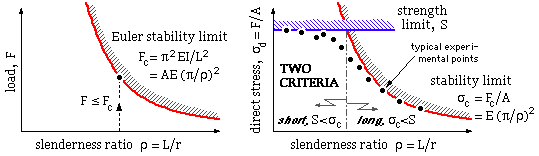If a squat barrel is compressed then buckling is impossible, and failure occurs when the load- induced stresses reach the strength   S of the material. This additional failure mechanism is indicated in the   Two Criteria plot above in which the ordinates of the LH graph have been reduced by the cross- sectional area,   A, that is, transformed into direct compressive stress,   σd = F/A. The material strength   S acts as a bound additional to the Euler stability limit   σc = Fc /A, however typical experimental failure points lie below both limits. The plot shows clearly that   σd cannot exceed either   σc or   S.

Historically, columns have been classed as either :
 short - which fail when the strength limit   S is reached (a material criterion), or long - which fail when the stability limit   σc is reached (a geometric criterion) . . . often described by empirical design equations such as the   'parabolic' or   'JB Johnson' formulae.
Such a distinction will be found unnecessary with the rational approach adopted here which recognises the interaction between the two criteria -   stability and   strength. The approach is based on the concept of imperfect columns.

Perfect columns, like perfect mechanisms, are theoretical idealities. Imperfections must arise in practice - and the effect of these is invariably to reduce the load carrying capacity predicted by elementary idealised theory. We examine a practical column whose imperfections prior to load application may be modelled by either of the two arrangements illustrated below, in which the imperfection is characterised by the maximum eccentricity   emax.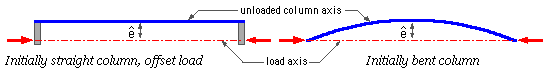Although such non- Eulerian initial conditions may be quite deliberate, as in the case of a walking stick, we shall for convenience refer to them as   'imperfections'.

Imperfect columns are not unlike beams in that bending is a major failure mechanism, however there are two significant differences between columns and beams :-

• Beam loading geometry is essentially invariant when loads are applied ( deflections are small and do not affect bending moments to a significant degree ) whereas the bending moments in an imperfect column are affected by bending deflections. This last phenomenon causes non-linear behaviour ( stresses and deflections are NOT proportional to load ) and geometric instability.
• Beams are usually subjected only to bending, with direct stresses for the most part negligible. Columns are loaded in bending plus direct compression - one of the few situations where direct effects cannot be neglected in the presence of bending. The maximum stress in an imperfect column is a combination of direct and bending stresses, and the column is assumed to be on the point of failure when this maximum stress reaches the strength of the material.
The variables which enter into the analysis are reviewed in this schema :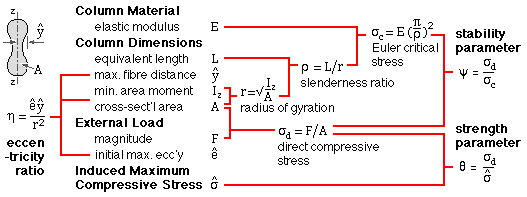The variables are condensed conveniently into three dimensionless groups :
• The   eccentricity ratio η, reflects the relative severity of the imperfections .
• The   strength parameter θ, is the ratio of the direct compressive stress to the maximum compressive stress ( direct + bending ) which equals the strength   S of the material at failure. The strength parameter is a measure of tendency towards strength failure - the parameter cannot exceed unity.
• The   stability parameter ψ, is the ratio of the direct compressive stress to the critical buckling ( Euler) stress. The stability parameter is a measure of tendency towards stability failure - the parameter cannot exceed unity.
As the three dimensionless groups embody load, strength, dimensional and safety aspects, the design equation sought will be of the form :   function ( η, θ, ψ ) = 0. The equation is derived from beam deflection theory rather than by energy methods.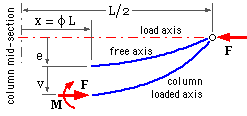A horizontal column is symmetric about its midsection   x = 0.
A free body of its right hand end is shown, with boundary at   x = φL where   -1/2 ≤ φ ≤ 1/2. The stress resultant at the free body's LH boundary consists of the axial force,   F ( which equals the column load ) and the bending moment,   M. The eccentricity of the unloaded column axis from the load's line of action is   e - the relationship between   e and   x will depend upon the form of initial imperfection which exists. Three different configurations, each characterised by maximum eccentricity   emax, are examined in parallel :
 Configuration : straight; offset load bent parabolically bent sinusoidally ( a) e   at φ = emax   (constant) emax ( 1 - 4φ2 ) emax cos πφ For equilibrium of the free body, in which   v is the additional deflection at   φ when the load   F is applied ( b) M   =   F ( e + v )     and   =   - EI d2v/dx2     from bending theory. Equating these right hand sides, substituting   x = φL and noting that   FL2/EI = π2 ψ where   ψ is a constant common to all cross-sections, yields ( c) d2v/dφ2 + π2 ψ v   =   - π2 ψ e     whose solution is of the form ( d) v   =   C cos( π√ψ.φ ) + D sin( π√ψ.φ ) + particular integral . . . in which   C and   D are integration constants, and the particular integral refers to the relevant equation ( a) for   e on the RHS of ( c). The integration constant   D must vanish by symmetry about   φ = 0, and   C must satisfy zero deflection at the ends   (   v=0 at   φ=±1/2 ). Inserting these boundary conditions, the elastic curves for the three cases are ( e) v / emax = sec(π/2√ψ) cos(π√ψ.φ) - 1 8/π2ψ sec(π/2√ψ) cos(π√ψ.φ) - 1 - 8/π2 ψ + 4φ2 [ ψ/(1-ψ)] cos π φ The relevant maximum deflection at the centre of the column,   φ = 0, follows as ( f) vmax /emax = sec(π/2√ψ) - 1 8/π2 ψ [ sec(π/2√ψ) - 1 ] - 1 ψ/(1-ψ) The maximum compressive stress, on the concave face of the column at   φ = 0 is ( g) σmax   =   σdirect + σbending max                           - which with ( b) becomes                       =   σd + F ( emax + vmax ) ymax / Iz     - or, normalising by σd         1/θ       =   1 + η ( vmax /emax + 1 ) Substituting the appropriate ratio   vmax /emax from ( f) into this last gives the desired relationships for the three cases ( h) 1/θ = 1 + η sec(π/2√ψ) 1 + η 8/π2 ψ [ sec(π/2√ψ) - 1 ] 1 + η / ( 1 - ψ) . . . where   θ = σd /σmax ,   ψ = σd /σc   and both   θ, ψ ≤ 1.The strength-stability interaction equations ( h) are plotted here as loci corresponding to constant values of eccentricity ratio.
The leftmost equation corresponding to straight columns with offset loads is known as the   Secant Equation and appears as dashed blue loci in the plot.

The full red loci refer to both the initially bent columns - there is no difference distinguishable at the scale of the graphs between parabolic and sinusoidal imperfections. This reinforces the previous conclusion that an imperfection's actual form is of lesser significance than its magnitude. It is justifiable therefore to adopt the simplest equation here - for the column initially bent sinusoidally - to represent all forms of imperfection. This also has the great advantage of enabling closed form solutions. So, substituting for   ψ, θ in the rightmost equation ( h)

( j)         ( σmax - σd ) ( σc - σd )   =   η σd σc   ;       σd ≤ σc, σmax

from which the maximum stress   σmax may be found for a given load (implicit in the direct stress   σd) acting on an imperfect column which is characterised by a certain critical Euler stress   σc and eccentricity ratio   η.

If the   load safety factor,   n, corresponds to a certain actual load,   F, then the direct stress at failure,   σd = Ffailure /A = n F/A, corresponds to   σmax reaching the material's compressive strength,   S. Application of ( j) to this failure situation yields the design equation :-

( 2)         σd2 - σd [ S + ( 1 + η ) σc ] + S σc   =   0   ;       σd = n F/A   ≤ σc , S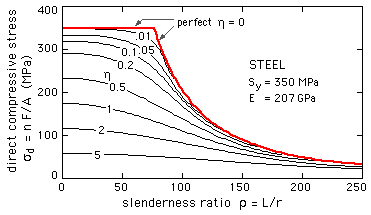The direct stress from ( 2) is plotted here for a particular steel. The graph demonstrates the generality that   the larger the imperfections, the smaller the load capacity.

The intercepts on the direct stress axis are   S/( 1+η ), since for a short squat column   ρ --> 0, instability tendencies are negligible, σc --> ∞, and so the intercepts follow from ( 2) or more immediately from the sketch of the short column below.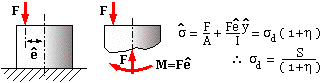If the column is brittle then the tensile stress on the convex side, evaluated along the lines of ( g), may be more significant than the larger compressive stress which must be the more critical peak for ductiles.

Safety factor is a factor on LOAD
- ALWAYS -

When stress is proportional to load then the safety factor applies equally well to stresses. But column stresses are NOT proportional to load, as this example illustrates.

Column   design is more complex than this example suggests. Cross-sectional geometric properties occur in all terms of ( 2) except the material strength, so design requires a trial-and-error approach.
A more fundamental difficulty in applying ( 2) however, is deciding on what value of eccentricity to use when imperfections are nominally absent - the column is perceived as perfect but manufacture and assembly must introduce   some imperfections. We would expect that the longer and thinner the column, the greater would be the difficulty in ensuring perfection, so :-
maximum eccentricity   emax or eccentricity ratio   η   =
some increasing function of length   L or of slenderness ratio   ρ

Various proposals ( based upon observations of course ) have appeared in the literature, however the recommendations of the Steel Structures Code will be adopted here. This Code implements equation ( 2) with a safety factor of 5/3, and stipulates that :

( k)         η     ρ2   =   3 E-5 ∗ ρ2   =   3 E-5 ( π2 E/σc )     and =   ση c

. . . . where the   equivalent imperfection stress   ση ≡ 3 E-5 π2 E is evidently a material characteristic, say   60 MPa for steel. A lower value is probably permissible for small non-critical components in mechanical engineering.

Equations ( 2) and ( k) lead to the more useful design equation applicable to   nominally perfect columns, namely :-

( 3)         σd2 - σd ( S + σc + ση ) + S σc   =   0   ;       σd = n F/A   ≤   σc , S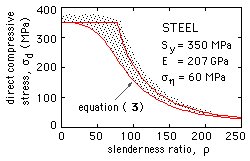The direct stress at failure ( n = 1) according to this equation, forms a lower bound to experimental results from tests on ostensibly perfect columns. The scatter of these results is due to a number of causes which the foregoing treatment neglects, among which are plastic behaviour, non-ideal supports, buckling mode interaction (especially local buckling) and residual stresses.
Residual stresses arise when a complex section cools differentially after manufacture by hot rolling, forging, welding, etc. and may typically reach 30-40% of yield. They are thus far from negligible, and heat treatment aimed at reducing them is usually impracticable, for structural sections at least. They may be partially allowed for when applying the above theory, by reducing the design yield strength accordingly.
The causes of scatter may be regarded as equivalent imperfections, and the Code's recommendations are presumably based on this all-embracing view.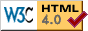Copyright 1999-2005 Douglas Wright,   doug@mech.uwa.edu.au
last updated May 2005